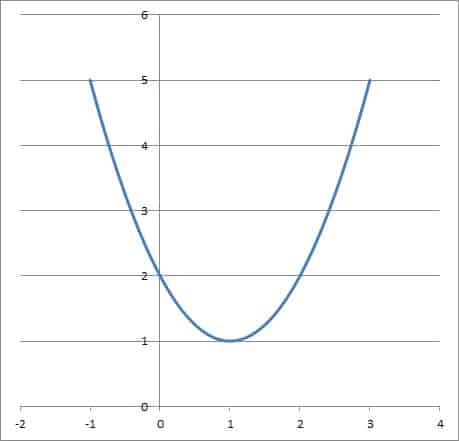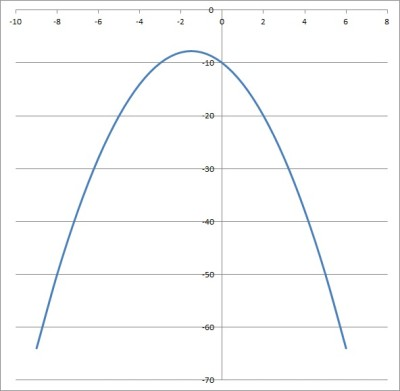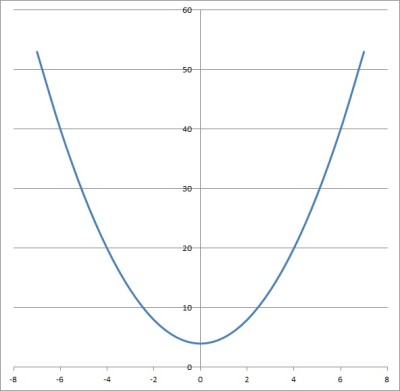# When Does A Quadratic Have No Solution? (3 Ways To Tell)

When working with quadratic equations, we often see one or two real solutions.  However, it is also possible that a quadratic will have no real solution.

So, when does a quadratic have no solution?  A quadratic equation has no solution when the discriminant is negative.  From an algebra standpoint, this means b2 < 4ac.  Visually, this means the graph of the quadratic (a parabola) will never touch the x axis.

Of course, a quadratic that has no real solution will still have complex solutions.

In this article, we’ll talk about how you can tell that a quadratic has no solution.  We’ll also look at some examples, along with how to write a quadratic equation given its complex solutions.

Let’s begin.

Having math trouble?

Looking for a tutor?

## When Does A Quadratic Have No Solution?

There are a few ways to tell when a quadratic equation has no solution:

• Look at the discriminant – if it is negative, there is no real solution to the quadratic.
• Look at the graph – if the parabola never touches the x-axis, there is no real solution to the quadratic.
• Look at the coefficients – there are some special cases that will tell you when there is no real solution to the quadratic (more on this later in the article!)

### Look At The Discriminant

The first way to tell if a quadratic has no real solution is to look at the discriminant. If the discriminant is negative, then the quadratic equation has no real solution.

Remember that for the quadratic equation given by:

• ax2 + bx + c = 0

the discriminant is the expression given by:

• b2 – 4ac

To get a negative discriminant, we need:

• b2 – 4ac < 0

or

• b2 < 4ac

Here is one example of a quadratic equation with no real solution:

•  x2 + 2x + 5 = 0

In this case, a = 1, b = 2, and c = 5.  This gives us:

• b2
• = (2)2
• = 4

and

• 4ac
• = 4(1)(5)
• = 20

So, b2 < 4ac (since 4 < 20), and thus the discriminant is negative.  This means that the quadratic has no real solution.

However, the equation does have two complex solutions, -1 + 2i and -1 – 2i.  (You can verify these solutions by using the quadratic formula with a = 1, b = 2, and c = 5).

You can also verify these two solutions by using FOIL on (x – (-1 + 2i))(x – (-1 – 2i)) to get back the original quadratic,  x2 + 2x  + 5.  (Remember that i2 = -1).

You can see the graph of this function below.

If you look closely, you will notice something interesting about the graph above: it never touches the x-axis (that is, the parabola never crosses the line y = 0).

Let’s take a closer look at why this is the case.

### Look At The Graph

Another way to tell if a quadratic has no real solution is to look at its graph.  For any quadratic equation, the graph will be a parabola.

Remember that one of the key features of a parabola is its vertex.  The vertex of a parabola is sort of like the “mountain top” (for negative values of a) or “valley bottom” (for positive values of a).As you can see, the quadratic function f(x) = x2 – 2x + 2 is a parabola that never touches the x axis, since the vertex (valley bottom) lies above x axis at x = 1. This means the quadratic equation has no real solution.

Note that a quadratic with no real solution could have a graph that is completely below the x axis (in that case, one of the conditions is that a < 0). An example is below:As you can see, the quadratic function f(x) = -x2 – 3x – 10 is a parabola that never touches the x axis, since the vertex (mountain top) lies below x axis at x = -3/2. This means the quadratic equation has no real solution.

As you can see in the first graph pictured above, the parabola does not touch the x axis. This means the quadratic equation x2 – 2x + 2 = 0 has no real solution.

Instead, it has two complex solutions: 1 + i and 1 – i.

Be careful: for a quadratic equation to have no real solution, its graph must never touch the x axis.  If the graph touches the x axis at all, then it has either one (repeated) real solution or two distinct real solutions.

Having math trouble?

Looking for a tutor?

### Look At The Coefficients

You can also look at the coefficients of a quadratic equation in standard form to tell if it has no real solution.

Remember that the standard form of a quadratic equation has zero on one side, and terms in descending order on the other:

• ax2 + bx + c = 0

There is a special case that will tell us if a quadratic has no real solution: if b = 0 when a and c share the same sign (both positive or both negative), then there will be no real solution.

The quadratic formula becomes much simpler when b = 0.  After simplifying, we find that the solutions are the positive and negative square roots of –c / a.

If c and a are both positive, then c / a is positive, and –c / a is negative.

Likewise, if c and a are both negative, then c / a is positive, and –c / a is negative.

In either of those cases, we are taking the square root of a negative, which gives us two complex solutions to the quadratic (that is, no real solution).

For example, the quadratic equation x2 + 4 = 0 has no real solution.  In this case, a = 1, b = 0, and c = 4.

Since b = 0 when a and c have the same sign (both are positive), we know there is no real solution.  In fact, the solutions are 2i and -2i (you can verify this with the quadratic formula).

We can also verify this if we FOIL (x – 2i)(x + 2i), cancel like terms, and use i2 = -1 to simplify to get back to x2 + 4.

Similarly, the quadratic equation -2x2 – 18 has no real solution.  In this case, a = -2, b = 0, and c = -18.

Since b = 0 when a and c have the same sign (both are negative), we know there is no real solution.  In fact, the solutions are 3i and -3i.

Remember that you can always use a calculator to help you verify the solutions to a quadratic equation.  You can also use a quadratic equation solver, such as this one from WolframAlpha.

For WolframAlpha’s calculator, remember that:

• The quadratic coefficient (x2 coefficient) means a
• The linear coefficient (x coefficient) means b
• The constant coefficient means c

## Examples Of Quadratic Equations With No Real Solution

Here are some examples of quadratic equations with no real solution.  Look at them to see if you notice a pattern before reading further.

• x2 + x + 1 = 0
• x2 + x + 2 = 0
• x2 + x + 3 = 0
• x2 + x + 4 = 0
• x2 + x + 5 = 0

One thing you might notice is that the x2 coefficients (a values) are all equal to 1.

Another thing you might notice is that the x coefficients (b values) are all equal to 1.

One last thing you might notice is that the constant terms (c values) are the sequence of whole numbers:

• 1, 2, 3, 4, 5

After we notice the pattern, we can easily create more of these quadratic equations with no real solution.  When we plug a = 1 and b = 1 into the quadratic formula, the discriminant simplifies to 1 – 4c.

As long as c is a positive number greater than ¼, we will get a quadratic with no real solution.  Any positive whole number is greater than ¼, so we can choose any of them for c.

We can also change the sign of all the b values to get an entire new set of quadratic equations with no real solution:

• x2 – x + 1 = 0
• x2 – x + 2 = 0
• x2 – x + 3 = 0
• x2 – x + 4 = 0
• x2 – x + 5 = 0

After plugging in a = 1 and b = -1 into the quadratic formula, the discriminant still simplifies to 1 – 4c.

Once again, as long as c is a positive number greater than ¼, we will get a quadratic with no real solution.

There are many other possibilities for quadratic equations with no real solutions.  All you need to do is choose a, b, and c so that the discriminant is negative (that is, b2 < 4ac).

## Does A Quadratic Equation Always Have A Solution?

A quadratic equation does not always have a real solution.  However, a quadratic equation always has a solution if we consider complex/imaginary numbers.

In terms of real solutions, there are always either 0, 1, or 2 real solutions to a quadratic equation, depending on the sign of the discriminant.

• If the discriminant is positive, there are exactly 2 real solutions.
• If the discriminant is zero, there is exactly one real solution (a repeated root).
• If the discriminant is negative, there are exactly 2 complex solutions (they are complex conjugates), with no real solution.

Remember that two complex conjugates have the form a + bi and a – bi.  In other words, they have the same real part, but opposite imaginary parts.

For example, 2 + 3i and 2 – 3i are complex conjugates.

## How Do You Write A Quadratic Equation With No Real Solution?

Now it’s time to work backwards.  Given a pair of complex conjugate solutions, we want to find a quadratic equation that has those solutions.

This is easy to do, once you know the method.  If the complex solutions are r and s, then all we need to do is FOIL (x – r)(x – s) to find the quadratic.

This would give us the quadratic equation x2 – (r + s)x + rs.  Let’s try an example.

### Example: Writing A Quadratic Equation With Given Complex Solutions (No Real Solution)

Assume we want to find a quadratic equation with roots 2i and -2i.  Then we have r = 2i and s = -2i.

The equation would be (x – r)(x – s) = 0 with r = 2i and s = -2i.  This gives us:

• (x – 2i)(x – (-2i)) = 0
• (x – 2i)(x + 2i) = 0

Using FOIL on the left side gives us x2 – 2ix + 2ix – 4i2 = 0  The like terms cancel to give us x2 – 4i2 = 0.

Since i2 = -1, we can simplify to x2 – 4(-1) = 0, or x2 + 4 = 0.

You can see the graph of the parabola y = x2 + 4 below (note that it never touches the x-axis).As you can see, the quadratic function f(x) = x2 + 4 is a parabola that never touches the x axis, since the vertex (valley bottom) lies above x axis at x = 0. This means the quadratic equation has no real solution.

## Conclusion

Now you know when a quadratic equation has no solution (in the real numbers).  You also know what to look out for in terms of the discriminant, the graph, and the coefficients.